Question

# Two long parallel wires are 3.2 cm apart from one another. the current in wire A...

Two long parallel wires are 3.2 cm apart from one another. the current in wire A is 5.8A, wire B is 7.2A borh into the page.

find magnitude and direction of the magnetic field at a point halfway between A and B. include diagram of set up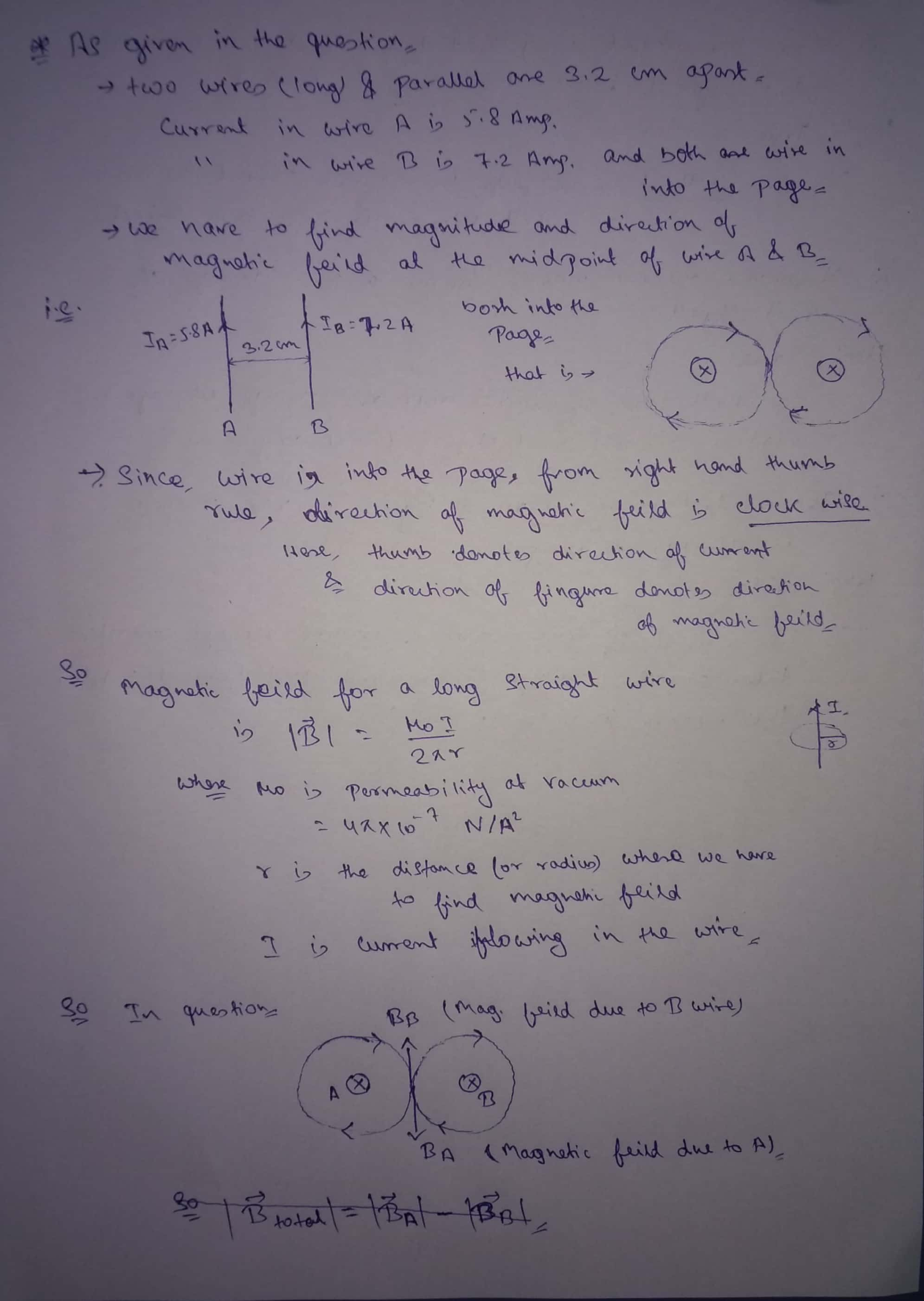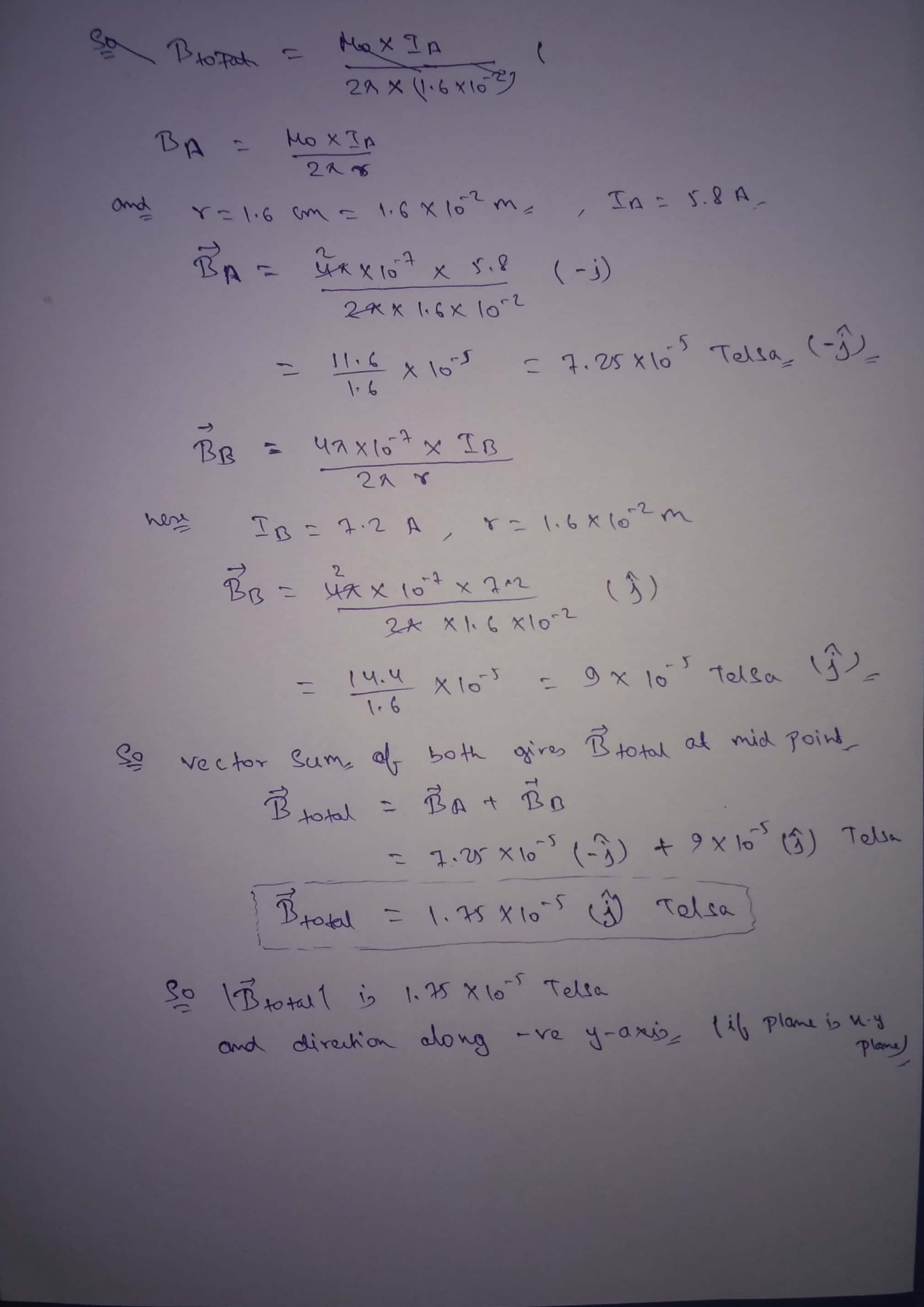#### Earn Coins

Coins can be redeemed for fabulous gifts.

Similar Homework Help Questions
• ### Two long, straight parallel wires are 10.0 cm apart and carry 3.20 A currents in the...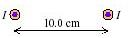Two long, straight parallel wires are 10.0 cm apart and carry 3.20 A currents in the same direction (see the figure). Find the direction of the magnetic field at point P1, midway between the wires. Find the magnitude of the magnetic field at point P1, midway between the wires. Find the direction of the magnetic field at point P2, 25.0 cm to the right of P1. Find the magnitude of the magnetic field at point P2, 25.0 cm to the...

• ### 1. Two long wires are parallel to one another and the y- axis. The wires are...

1. Two long wires are parallel to one another and the y- axis. The wires are separated by a distance of 15 cm apart. The wire on the left has a current of 2.5 A in the upward direction and the wire on the right has a current of 1.5 A in the upward direction. What is the magnetic field half way between the wires. A. 2.7 µT inward B. 10.7 µT outward C. 10.7 µT inward D. 2.7 µT...

• ### Two long, straight, parallel current-carrying wires are shown below. The wires are parallel to the (the...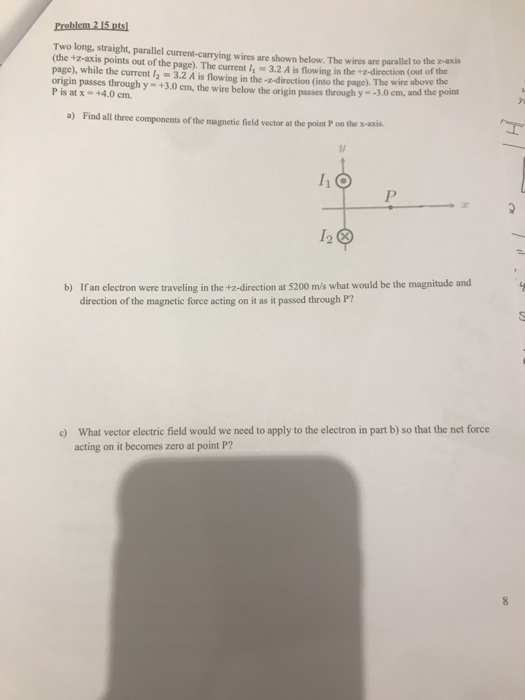Two long, straight, parallel current-carrying wires are shown below. The wires are parallel to the (the +z-axis points out of the page). The current 3.2 A is flowing in the +2-direction (out of the page), while the current 12-3.2 A is flowing in the z-direction (into the page). The wire abov he origin passes through y- +3.0 cm, the wire below the origin passes through y- -3.0 cm, P is atx +4.0 cm. a) Find all thrce components of the...

• ### (hrw8c29p68) Each of two long straight parallel wires 8 cm apart carries a current of 125...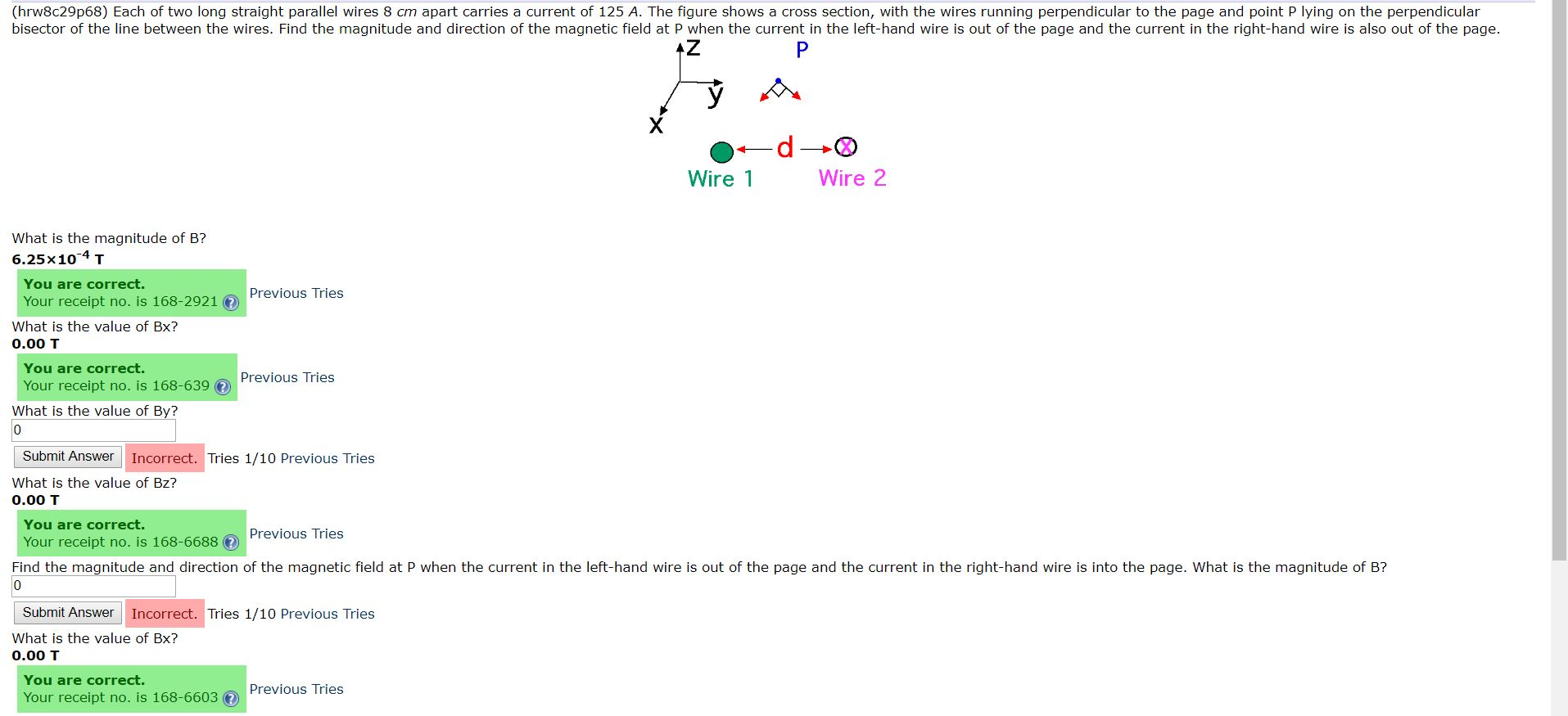(hrw8c29p68) Each of two long straight parallel wires 8 cm apart carries a current of 125 A. The figure shows a cross section, with the wires running perpendicular to the page and point P lying on the perpendicular bisector of the line between the wires. Find the magnitude and direction of the magnetic field at P when the current in the left-hand wire is out of the page and the current in the right-hand wire is also out of the...

• ### (hrw8c29p68) Each of two long straight parallel wires 13 cm apart carries a current of 150...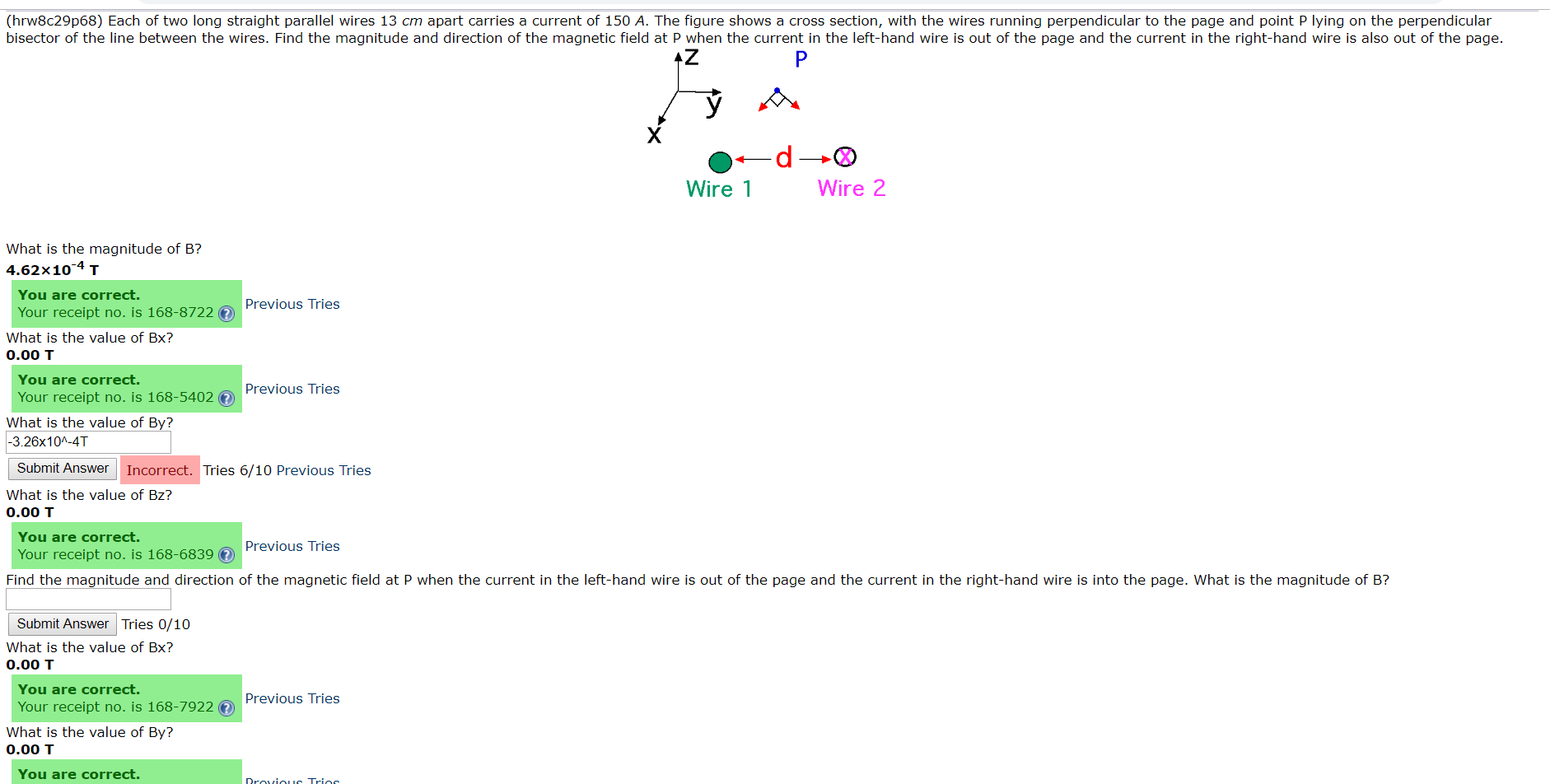(hrw8c29p68) Each of two long straight parallel wires 13 cm apart carries a current of 150 A. The figure shows a cross section, with the wires running perpendicular to the page and point P lying on the perpendicular bisector of the line between the wires. Find the magnitude and direction of the magnetic field at P when the current in the left-hand wire is out of the page and the current in the right-hand wire is also out of the...

• ### Two long parallel wires 8.20 cm apart carry the same current (given below) in the same...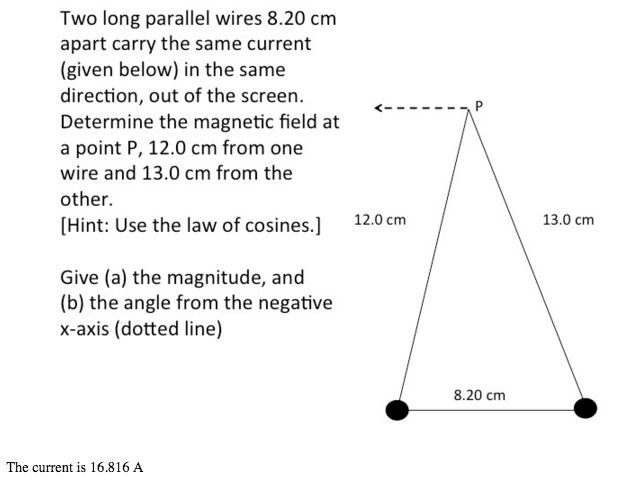Two long parallel wires 8.20 cm apart carry the same current (given below) in the same direction, out of the screen. Determine the magnetic field at a point P, 12.0 cm from one wire and 13.0 cm from the other. Give (a) the magnitude, and (b) the angle from the negative x-axis (dotted line) The current is 16.816 A

• ### Two long straight wires are parallel and 11 cm apart. They are to carry equal currents...

Two long straight wires are parallel and 11 cm apart. They are to carry equal currents such that the magnetic field at a point halfway between them has magnitude 440 �T. How much current (in A) is needed?

• ### Two long, straight, parallel wires are 10.0 cm apart and carry 5.00 A currents in the...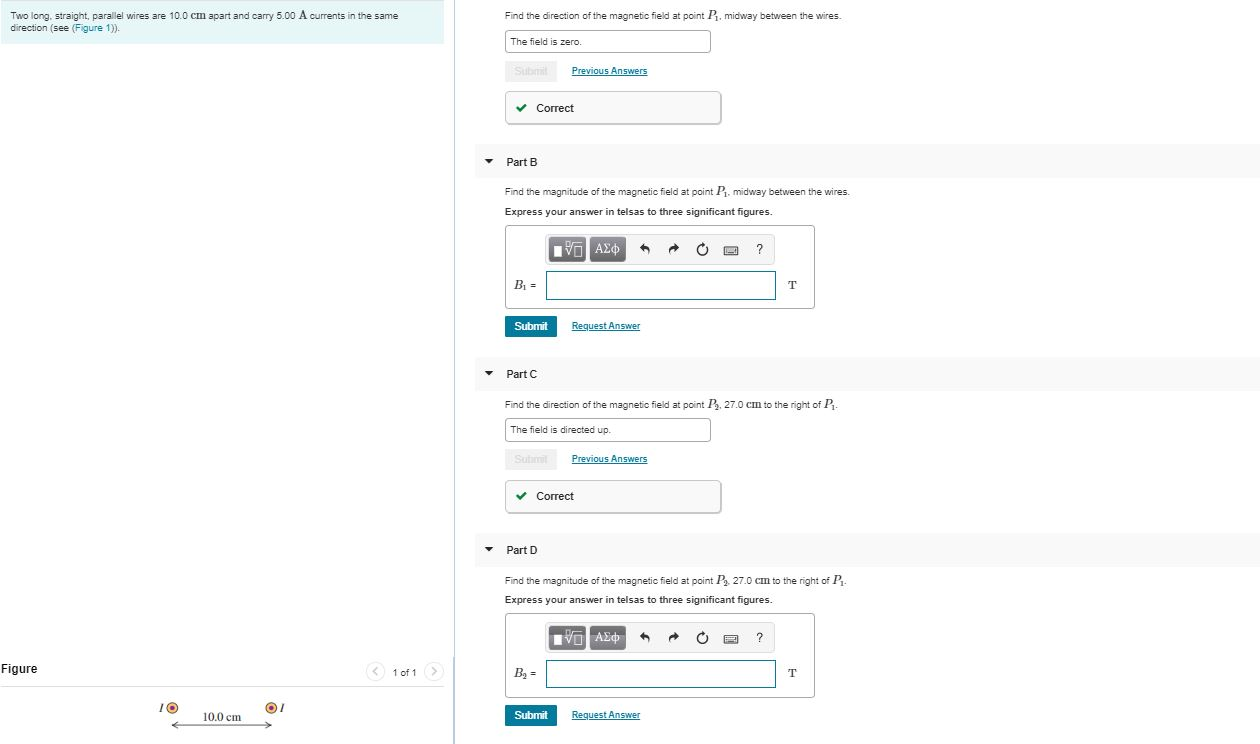Two long, straight, parallel wires are 10.0 cm apart and carry 5.00 A currents in the same direction (see Figure 1)). Find the direction of the magnetic field at point P.midway between the wires. The field is zero. Previous Answers Correct Part B Find the magnitude of the magnetic field at point P.midway between the wires. Express your answer in telsas to three significant figures. AED OR? B, Submit Request Answer Part C Find the direction of the magnetic field...

• ### i nerd help 5. Two long parallel wires 30.0 cm apart carry /,= 25A and 1,...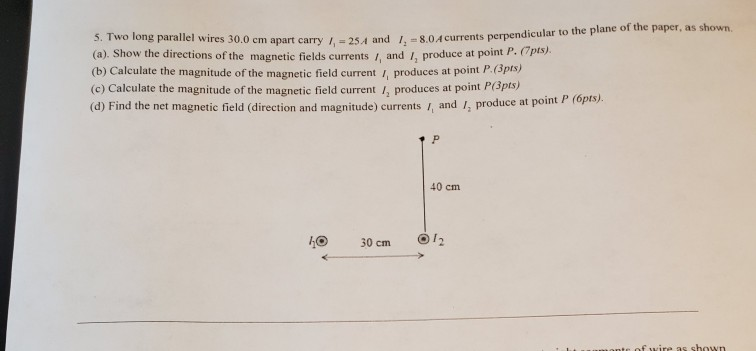i nerd help 5. Two long parallel wires 30.0 cm apart carry /,= 25A and 1, = 8.0 A currents perpendicu wires 30.0 cm apart carry = 25.4 and 804 currents perpendicular to the plane of the paper, as shown (a). Show the directions of the magnetic fields currents, and /, produce at point P.(pos. (b) Calculate the magnitude of the magnetic field current produces at point P. (spis) (c) Calculate the magnitude of the magnetic field current /, produces...

• ### Two long, straight wires are parallel and 42 cm apart. (a) If each wire carries a...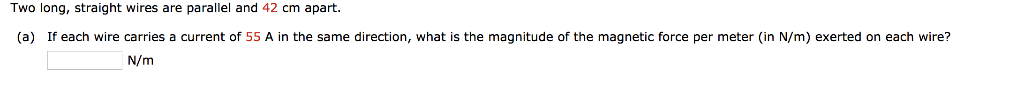Two long, straight wires are parallel and 42 cm apart. (a) If each wire carries a current of 55 A in the same direction, what is the magnitude of the magnetic force per meter (in N/m) exerted on each wire? ren